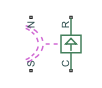# Reluctance Force Actuator

Magnetomotive device based on reluctance force

## Library

Magnetic Elements

•## Description

The Reluctance Force Actuator block models a generic magnetomotive device based on reluctance force.

The block is based on the following equations:

`$F=-0.5\cdot {\Phi }^{2}\cdot \frac{d\Re }{dx}$`
`$\Re \left(x\right)=\frac{x}{{\mu }_{0}\cdot {\mu }_{r}\cdot A}$`
`$u=dx$`

where

 F Reluctance force Φ Flux in the magnetic circuit $\Re$ Reluctance x Thickness or length of the air gap μ0 Permeability constant μr Relative permeability of the material A Cross-sectional area of the section being modeled u Velocity

Connections N and S are magnetic conserving ports, and connections C and R are mechanical translational conserving ports. The magnetic force produced by the actuator acts to close the gap, therefore the resulting force is negative when it acts from C to R.

### Variables

To set the priority and initial target values for the block variables prior to simulation, use the Initial Targets section in the block dialog box or Property Inspector. For more information, see Set Priority and Initial Target for Block Variables.

Nominal values provide a way to specify the expected magnitude of a variable in a model. Using system scaling based on nominal values increases the simulation robustness. Nominal values can come from different sources, one of which is the Nominal Values section in the block dialog box or Property Inspector. For more information, see Modify Nominal Values for a Block Variable.

## Basic Assumptions and Limitations

• The current excitation in the system is constant.

• Only axial reluctance is modeled.

## Parameters

Initial air gap

Thickness or length of air gap at the beginning of simulation. The default value is `2` mm.

Minimum air gap

Minimal value of air gap, with the reluctance force acting to close the air gap. The parameter value has to be greater than 0. The default value is `1e-4` mm.

Cross-sectional area

Area of the section being modeled. The default value is `0.01` m^2.

Relative permeability of material

Relative permeability of the section material. The default value is `1`.

Contact stiffness

Stiffness that models the hard stop at the minimum air gap position. The default value is `10e6` N/m.

Contact damping

Damping that models the hard stop at the minimum air gap position. The default value is 500 N/(m/s).

## Ports

The block has the following ports:

`N`

Magnetic conserving port associated with the block North terminal.

`S`

Magnetic conserving port associated with the block South terminal.

`R`

Mechanical translational conserving port associated with the rod.

`C`

Mechanical translational conserving port associated with the case.

## Version History

Introduced in R2010a